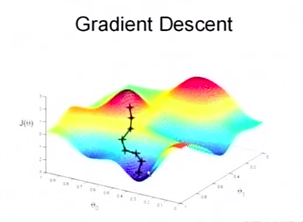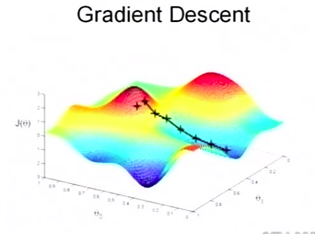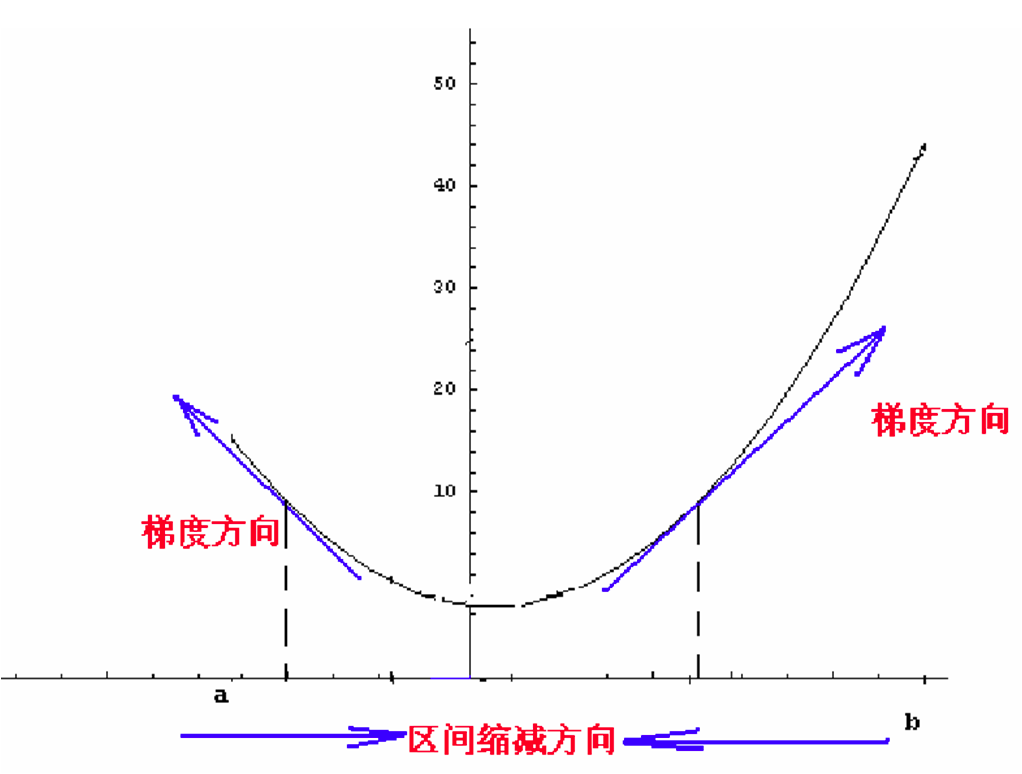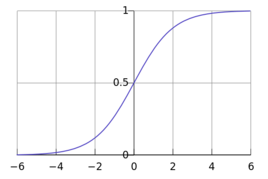# 回归问题

f(x)=wx+b$f(x) = wx+b$ 的形式。 ,然后估测w和b。

l(y;w,b)=i=0n(wxi+byi)2

## 最小二乘法

(f(x))w=i=1n2(wxi+byi)xi

(f(x))b=i=1n2(wxi+byi)

## 梯度下降法f(x)=limΔx0f(x0+Δx)f(x0)Δx=limxx0f(x)f(x0)xx0w=wiαf(w,b;x)w

w=wiαi=1n2(wxi+byi)xi

# Logistic回归

f(x)={10 if x is True otherwise【我估计发明者就是凑出来的】

f(x)=11+ex

# 0-1 损失函数(Zero-One Loss)

，则损失函数可定义为：

0,1=i=0|D|If(x(i))y(i)

Ix={10 if x is True otherwise

zero_one_loss = T.sum(T.neq(ye, y))

ye表示估计值，y是真实值。程序的表示似乎比数学简明多了。

# 负对数似然损失 (Negative Log-Likelihood Loss,NLL)

p{yi=1|xi}=f(xi)

D={(x(0),y(0)),(x(1),y(1)),...,(x(k),y(k))}

L(θ;D)=i=0kf(y=y(i)|x(i),θ)

L(θ;D)=i=0klogf(y=y(i)|x(i),θ)

C(θ;D)=i=0klogf(y=y(i)|x(i),θ)

# 梯度下降与随机梯度下降

C(θ;D)=i=0kC(θ;x(i),y(i))

C(θ;x(i),y(i))=logf(y=y(i)|x(i),θ)1. 批量梯度下降—最小化所有训练样本的损失函数，使得最终求解的是全局的最优解，即求解的参数是使得风险函数最小。

1. 随机梯度下降—最小化每条样本的损失函数，虽然不是每次迭代得到的损失函数都向着全局最优方向， 但是大的整体的方向是向全局最优解的，最终的结果往往是在全局最优解附近。

# softmax回归

hθ(x)=11+exp(θTx),

J(θ)=1m[i=1my(i)loghθ(x(i))+(1y(i))log(1hθ(x(i)))]

P(Y=i|x,W,b)=softmaxi(Wx+b)=eWix+bijeWjx+bj

ypred=argmaxiP(Y=i|x,W,b)